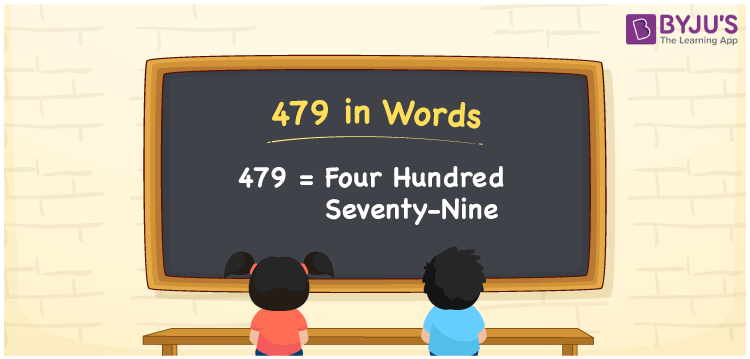# 479 in Words

479 in words is written as Four hundred seventy-nine. In both the International System of Numerals and the Indian System of Numerals, 479 is written as Four hundred seventy-nine. The number 479 is a Cardinal Number as it represents some quantity. For example, “there are 479 passengers, travelling daily through this route”.

 479 in Words Four hundred seventy-nine Four hundred seventy-nine in Number 479

## 479 in English Words

We write 479 in English Words using the letters of the English alphabet. Therefore, we read 479 in English as “Four hundred seventy-nine.”## How to Write 479 in Words?

To write 479 in words, we shall use the place value chart. In the place value chart, write 4 in the hundreds, 7 in the tens, and 9 in the ones, respectively. Now let us make a place value chart to write the number 479 in words.

 Hundreds Tens Ones 4 7 9

Thus, we can write the expanded form as

4 × Hundred + 7 × Ten + 9 × One

= 4 × 100 + 7 × 10 + 9 × 1

= 400 + 70 + 9

= 479

= Four hundred seventy-nine.

479 is a natural number, the successor of 478 and the predecessor of 480.

479 in words – Four hundred seventy-nine

• Is 479 an odd number? – Yes
• Is 479 an even number? – No
• Is 479 a perfect square number? – No
• Is 479 a perfect cube number? – No
• Is 479 a prime number? – Yes
• Is 479 a composite number? – No

## Frequently Asked Questions on 479 in Words

Q1

### How to write 479 in words?

479 in words is written as Four hundred seventy-nine.
Q2

### How to write 479 in the International and Indian System of Numerals?

In both, the system of numerals, 479 in words, is written as Four hundred seventy-nine.
Q3

### What is the preceding number of 479?

The number that precedes 479 is 478.